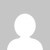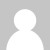# Entropy and Information Gain in Decision Tree Learning

[wptelegram-join-channel]

## Explain the concepts of Entropy and Information Gain in Decision Tree Learning.

While constructing a decision tree, the very first question to be answered is, Which Attribute Is the Best Classifier?

The central choice in the ID3 algorithm is selecting which attribute to test at each node in the tree.

We would like to select the attribute that is most useful for classifying examples.

What is a good quantitative measure of the worth of an attribute? We will define a statistical property, called information gain, that measures how well a given attribute separates the training examples according to their target classification.

## Video Tutorial

ID3 uses this information gain measure to select among the candidate attributes at each step while growing the tree.

ENTROPY MEASURES THE HOMOGENEITY OF EXAMPLES

Entropy characterizes the (im)purity of an arbitrary collection of examples.

Given a collection S, containing positive and negative examples of some target concept, the entropy of S relative to this boolean classification is

where p+, is the proportion of positive examples in S and p-, is the proportion of negative examples in S.

In all calculations involving entropy, we define 0log0 to be 0.

Let, S is a collection of training examples,

p+ the proportion of positive examples in S

p the proportion of negative examples in S

Examples

Entropy (S) =  – p+ log2 p+ plog2 p–      [0 log20 = 0]

Entropy ([14+, 0–]) = – 14/14 log2 (14/14) –  0 log2 (0) = 0

Entropy ([9+, 5–])  = – 9/14 log2 (9/14) –  5/14 log2 (5/14) = 0,94

Entropy ([7+, 7– ])  = –  7/14 log2 (7/14) –  7/14 log2 (7/14) =           = 1/2 + 1/2 = 1

INFORMATION GAIN MEASURES THE EXPECTED REDUCTION IN ENTROPY

Given entropy as a measure of the impurity in a collection of training examples, we can now define a measure of the effectiveness of an attribute in classifying the training data.

Now, the information gain is simply the expected reduction in entropy caused by partitioning the examples according to this attribute.

More precisely, the information gain, Gain(S, A) of an attribute A, relative to a collection of examples S, is defined as,

where Values(A) is the set of all possible values for attribute A, and S, is the subset of S for which attribute A has value v (i.e., S_v= {s ∈ S|A(s) = v})

For example, suppose S is a collection of training-example days described by attributes including Wind, which can have the values Weak or Strong.

Information gain is precisely the measure used by ID3 to select the best attribute at each step in growing the tree.

The use of information gain is to evaluate the relevance of attributes.

### Solved Numerical Examples and Tutorial on Decision Trees Machine Learning:

1. How to build a decision Tree for Boolean Function Machine Learning

2. How to build a decision Tree for Boolean Function Machine Learning

3. How to build a Decision Tree using ID3 Algorithm – Solved Numerical Example – 1

4. How to build a Decision Tree using ID3 Algorithm – Solved Numerical Example -2

5. How to build a Decision Tree using ID3 Algorithm – Solved Numerical Example -3

6. Appropriate Problems for Decision Tree Learning Machine Learning Big Data Analytics

7. How to find the Entropy and Information Gain in Decision Tree Learning

8. Issues in Decision Tree Learning Machine Learning

9. How to Avoid Overfitting in Decision Tree Learning, Machine Learning, and Data Mining

10. How to handle Continuous Valued Attributes in Decision Tree Learning, Machine Learning

## Summary

This tutorial discusses, what are appropriate problems for Decision tree learning. If you like the tutorial share it with your friends. Like the Facebook page for regular updates and the YouTube channel for video tutorials.

### 2 thoughts on “Entropy and Information Gain in Decision Tree Learning”

1.Solve 18cs71 question paper 14-02-2022 module 3 id3 problem sir….

2.Sir we need this pdf pls…